## Introduction¶

In my last post I introduced a simple linear time-series model using indicator functions for forecasting. In the meantime, I was experimenting with some other ideas for non-complex models with good predictive power. One approach that has been on my mind for quite long now, is commonly known to Data Scientists as binning. A Statistician might be more familar with the name discretization or reducing the scale level of a random variable from numerical to categorical. An advantage of this technique is the reduction of noise - however, this comes at the cost of losing quite an amount of information.

In :
import warnings
warnings.filterwarnings("ignore")

import matplotlib.pyplot as plt
import numpy as np
import pandas as pd
from sklearn.naive_bayes import *
from sklearn.linear_model import LinearRegression


## Dataset¶

Since this is another time-series forecasting model, I decided to stick with the passengers dataset from last time. I didn't use the shampoo dataset because the size of this dataset seemed to be too small for the model to achieve acceptable predictive power.

In :
data = pd.read_csv("passengers.csv",index_col = 0).iloc[:,0]

In :
plt.figure(figsize = (12,6))
plt.plot(data)

Out:
[<matplotlib.lines.Line2D at 0x7f1509e4b4e0>]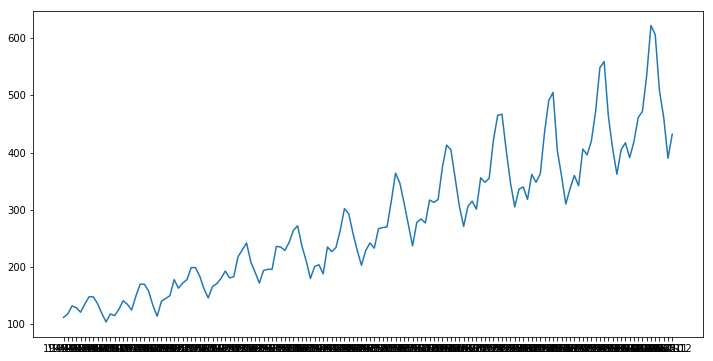In :
test_period = 36

train = data.iloc[:-test_period]
test = data.iloc[-test_period:]


## Preprocessing¶

Contrary to the last model, this approach cannot deal directly with non-stationary data so the trend in the data has to be removed via plain differencing.

In :
trend_removed = train.diff()

In :
plt.figure(figsize = (12,6))
plt.plot(trend_removed)

Out:
[<matplotlib.lines.Line2D at 0x7f15070ad198>]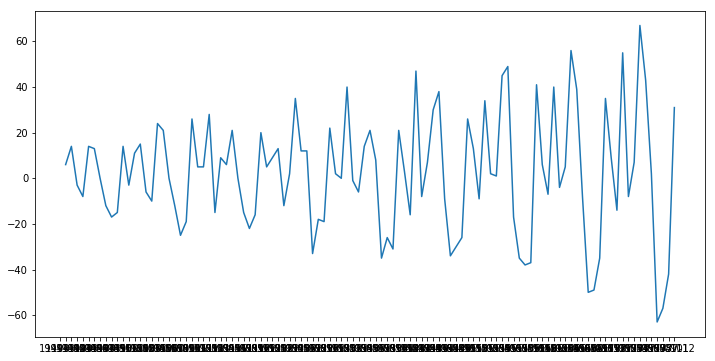As is visible in the plot of the differenced time-series, there is still a problem with heteroscedasticity which has to be solved as well. The variance of the dataset seems to be increasing over time, I assumed a linearly increasing variance:

$$\sigma^2_X(t)=t\cdot\sigma^2_X(1)=\sigma^2$$

If the assumption is correct, a simple division of $X$ by $\sqrt{t}$ should solve the problem:

In :
t_train = np.arange(len(train)).reshape(-1,1)
trend_removed = trend_removed / ((t_train+1)**(1/2)).reshape(-1)

plt.figure(figsize = (12,6))
plt.plot(trend_removed)

Out:
[<matplotlib.lines.Line2D at 0x7f15072f5908>]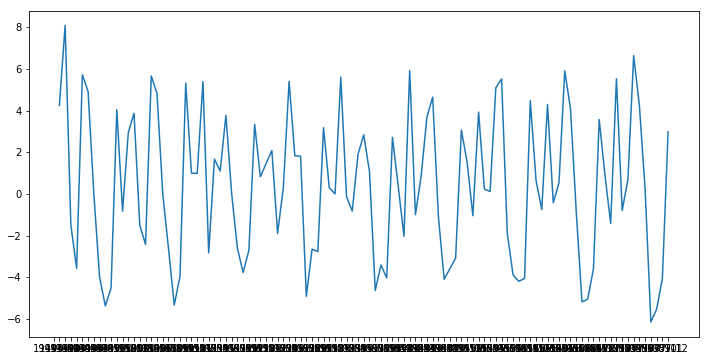The plot still looks a little non-stationary in the first few periods so I (arbitrarily) removed the first 5 datapoints as well.

In :
trend_removed = trend_removed.iloc[5:]


## Binning the data¶

To bin the data, $K\in\mathbb{Z^+}$ intervals on $\mathbb{R}$ are being assigned. Now, each continuous observation $x_t$ is replaced by an indicator $x_t^*=k,\quad k\in\left\{1;...;K\right\}$ where $k$ is the interval that $x_t$ falls in. While there are countless ways to define the intervals, I chose the intervals to be evenly spaced between $min(x_t)$ and $max(x_t)$. The number of intervals was chosen arbitrarily again - cross-validation could be applied here.

In :
n_bins = 10
bins = np.linspace(trend_removed.min(), trend_removed.max(), n_bins)
binned = np.digitize(trend_removed, bins)

binned_series = pd.Series(binned, index = trend_removed.index)


The mean of realizations $x_t$ in each interval is saved in a dictionary in order to map the interval category back to actual realizations.

In :
bin_means = {}

for binn in range(1,n_bins+1):
bin_means[binn] = trend_removed[binned == binn].mean()


To forecast future realizations, the classic approach of using $S\in\mathbb{Z}^+$ lagged realizations of $x_t^*$ will be applied. The amount of lags I chose was $12$, assuming that there is no longer auto-dependency of the process beyond a horizon of one year.

In :
lagged_list = []
for s in range(13):
lagged_list.append(binned_series.shift(s))

lagged_frame = pd.concat(lagged_list,1).dropna()

train_X = lagged_frame.iloc[:,1:]
train_y = lagged_frame.iloc[:,0]


Training regressors and regressands now look like this:

In :
train_X.head()

Out:
1 2 3 4 5 6 7 8 9 10 11 12
Month
1950-06 3.0 4.0 8.0 7.0 4.0 8.0 2.0 1.0 2.0 5.0 8.0 9.0
1950-07 9.0 3.0 4.0 8.0 7.0 4.0 8.0 2.0 1.0 2.0 5.0 8.0
1950-08 8.0 9.0 3.0 4.0 8.0 7.0 4.0 8.0 2.0 1.0 2.0 5.0
1950-09 5.0 8.0 9.0 3.0 4.0 8.0 7.0 4.0 8.0 2.0 1.0 2.0
1950-10 3.0 5.0 8.0 9.0 3.0 4.0 8.0 7.0 4.0 8.0 2.0 1.0
In :
train_y.head()

Out:
Month
1950-06    9
1950-07    8
1950-08    5
1950-09    3
1950-10    1
Name: 0, dtype: int64

To calculate the 'class'-means from before, I wrote a quick function that takes the predicted class as an input and returns the corresponding means.

In :
def get_mean_from_class(prediction):

return(bin_means[prediction])


## Predictive Model¶

Since we are now dealing with a categorical variable, Naive Bayes looked like a reasonable and interesting model to try out - especially since the is no need to create dummy variables for the sklearn implementation. Interestingly, Bernoulli Naive Bayes produced non-sensical predictions although the regressors (train_X) make much more sense to assume as categorical variables. Using Gaussian Naive Bayes was much better, probably because there is still a natural order in the regressors - that's why I recommend to experiment with a large variety of models, even though some assumptions might not be met perfectly.

In :
model = GaussianNB()

In :
model.fit(train_X, train_y)

Out:
GaussianNB(priors=None)
In :
pred_insample = model.predict(train_X)
pred_insample = pd.DataFrame(pred_insample, index = train_y.index)

resulting_prediction = pd.Series(np.nan, index = train_y.index)
for row in range(len(pred_insample)):
resulting_prediction.iloc[row] = get_mean_from_class(pred_insample.values[row])

In :
plt.figure(figsize = (12,6))
plt.plot(trend_removed)
plt.plot(resulting_prediction)

Out:
[<matplotlib.lines.Line2D at 0x7f15056ded30>]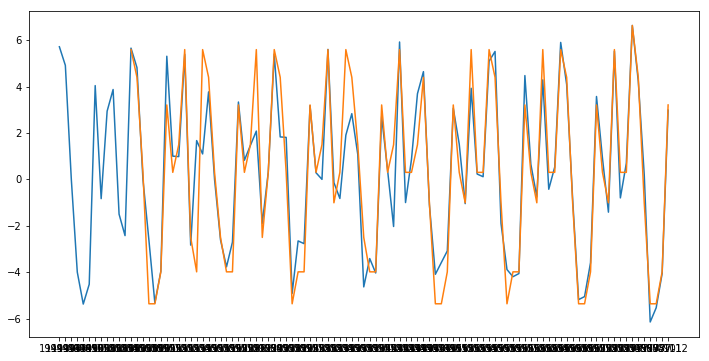The in-sample predictions are looking good despite the fact that there are actually only 10 possible prediction values.

Out-of-sample forecasts need to be calculated iteratively since lagged values are required.

In :
prediction_frame = pd.DataFrame(np.nan, index = test.index, columns = range(train_X.shape))
predictions = pd.Series(index = test.index)

prediction_frame.iloc[0,1:] = train_X.iloc[-1,:-1].values
prediction_frame.iloc[0,0] = train_y.iloc[-1]

In :
for i in range(len(test)):
pred = model.predict(prediction_frame.iloc[i,:].values.reshape(1,-1))
pred_num = get_mean_from_class(pred.reshape(-1))
predictions.iloc[i] = pred_num
try:
prediction_frame.iloc[i+1,1:] = prediction_frame.iloc[i,:-1].values
prediction_frame.iloc[i+1,0] = pred
except:
pass


Finally, the forecast has to be retransformed:

In :
trend_test = np.arange(len(train),len(train)+len(test)).reshape(-1,1)

final_prediction = predictions.cumsum()* ((trend_test+1)**(1/2)).reshape(-1)+train.iloc[-1]


## Results¶

In :
plt.figure(figsize = (12,6))
plt.plot(test)
plt.plot(final_prediction)

Out:
[<matplotlib.lines.Line2D at 0x7f15055c5668>]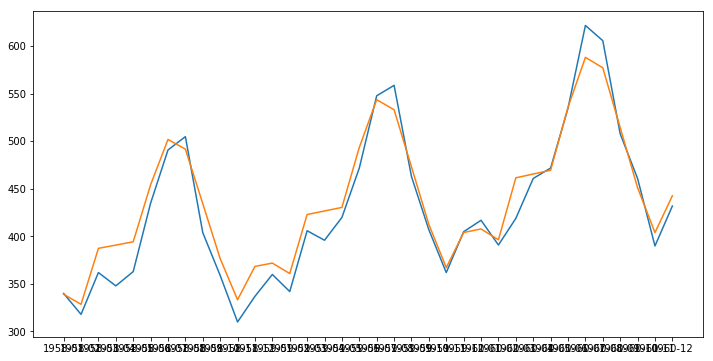In :
np.sqrt(np.mean((test-final_prediction)**2))

Out:
20.105172909688168

Pretty good result compared to the accuracy from last time. Nevertheless, there is still room for improvement, especially in terms of reducing the amount of selectable parameters in the "algorithm" - in that case the amount of bins could be selected automatically as this parameter is hard to choose properly. However, this post was just meant as a proof-of-concept prototype for this approach.

### 3 Comments

1.#### Victor

Coding like a Boss 😀

2.#### Neeraj Manandhar

Dear sir the article is great , but i am not able to get 100% out of it because the sample data set of ‘passengers dataset’ is not downloadable.
Can you please provide me with this sample data set so that , i can implement this idea in my data. Your help will be highly appreciated. Thank you in advance
manandharneeraj@gmail.com